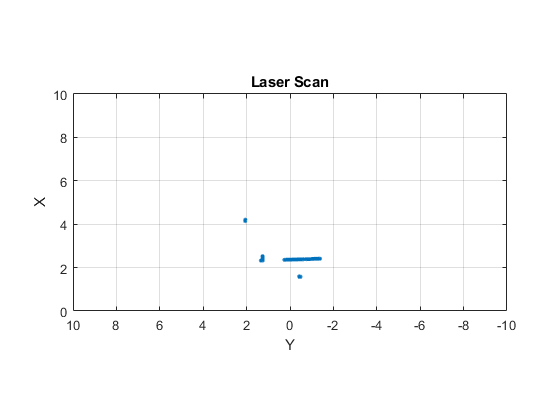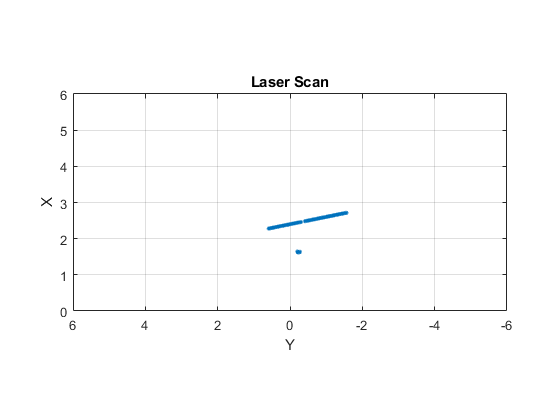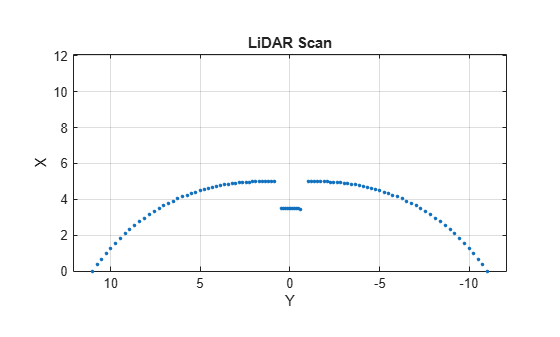# plot

Display laser or lidar scan readings

## Syntax

``plot(scanMsg)``
``plot(scanObj)``
``plot(___,Name,Value)``
``linehandle = plot(___)``

## Description

example

````plot(scanMsg)` plots the laser scan readings specified in the input `LaserScan` object message. Axes are automatically scaled to the maximum range that the laser scanner supports.```

example

````plot(scanObj)` plots the lidar scan readings specified in `scanObj`.```

example

````plot(___,Name,Value)` provides additional options specified by one or more `Name,Value` pair arguments.```
````linehandle = plot(___)` returns a column vector of line series handles, using any of the arguments from previous syntaxes. Use `linehandle` to modify properties of the line series after it is created.When plotting ROS laser scan messages, MATLAB® follows the standard ROS convention for axis orientation. This convention states that positive x is forward, positive y is left, and positive z is up. For more information, see Axis Orientation on the ROS Wiki.```

## Examples

collapse all

Connect to ROS network. Subscribe to a laser scan topic, and receive a message.

`rosinit('192.168.17.129')`
```Initializing global node /matlab_global_node_90279 with NodeURI http://192.168.17.1:50889/ ```
```sub = rossubscriber('/scan'); scan = receive(sub);```

Plot the laser scan.

`plot(scan)`Shutdown ROS network.

`rosshutdown`
```Shutting down global node /matlab_global_node_90279 with NodeURI http://192.168.17.1:50889/ ```

Connect to ROS network. Subscribe to a laser scan topic, and receive a message.

`rosinit('192.168.17.129')`
```Initializing global node /matlab_global_node_31712 with NodeURI http://192.168.17.1:51463/ ```
```sub = rossubscriber('/scan'); scan = receive(sub);```

Plot the laser scan specifying the maximum range.

`plot(scan,'MaximumRange',6)`Shutdown ROS network.

`rosshutdown`
```Shutting down global node /matlab_global_node_31712 with NodeURI http://192.168.17.1:51463/ ```

Specify lidar data as vectors of ranges and angles. These values include readings outside of the sensor range.

```x = linspace(-2,2); ranges = abs((1.5).*x.^2 + 5); ranges(45:55) = 3.5; angles = linspace(-pi/2,pi/2,numel(ranges));```

Create a lidar scan by specifying the ranges and angles. Plot all points of the lidar scan.

```scan = lidarScan(ranges,angles); plot(scan)```Remove invalid points based on a specified minimum and maximum range.

```minRange = 0.1; maxRange = 7; scan2 = removeInvalidData(scan,'RangeLimits',[minRange maxRange]); hold on plot(scan2) legend('All Points','Valid Points')```## Input Arguments

collapse all

`sensor_msgs/LaserScan` ROS message, specified as a `LaserScan` object handle.

Lidar scan readings, specified as a `lidarScan` (Navigation Toolbox) object.

### Name-Value Pair Arguments

Specify optional comma-separated pairs of `Name,Value` arguments. `Name` is the argument name and `Value` is the corresponding value. `Name` must appear inside quotes. You can specify several name and value pair arguments in any order as `Name1,Value1,...,NameN,ValueN`.

Example: `"MaximumRange",5`

Parent of axes, specified as the comma-separated pair consisting of `"Parent"` and an axes object in which the laser scan is drawn. By default, the laser scan is plotted in the currently active axes.

Range of laser scan, specified as the comma-separated pair consisting of `"MaximumRange"` and a scalar. When you specify this name-value pair argument, the minimum and maximum x-axis and the maximum y-axis limits are set based on a specified value. The minimum y-axis limit is automatically determined by the opening angle of the laser scanner.

This name-value pair works only when you input `scanMsg` as the laser scan.

## Outputs

collapse all

One or more chart line objects, returned as a scalar or a vector. These are unique identifiers, which you can use to query and modify properties of a specific chart line.